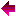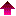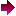Coordinate Systems Developer's Guide The Document and Graphics Classes

# Curve Objects

A curve object represents a single continuous curve. A curve is a sequence of segments with each segment starting at the end point of the preceding segment (except the first segment, of which only the end point is used as the start point of the second segment).

There are two kinds of segments, line segments and bezier segments.

A line segment is defined by its end point, p, and it defines a straight line from the preceding segment's end point to p.

A bezier segment consists of an end point, p, and two auxiliary points, p1 and p2. It defines a bezier curve starting at the preceding segment's end point, approximating p1 and p2 and ending at p.

The end points of the segments are usually called nodes. There are two special properties associated with them.

The first property is the continuity. The continuity can be `angle', `smooth' or `symmetrical'. This results in constraints in the editor when the user modifies a bezier object's auxiliary points:

angle

No constraint.

smooth

The node, the auxiliary points p2 of the node's segment, and p1 of the following segment must be collinear.

symmetrical

The node, the auxiliary points p2 of the node's segment, and p1 of the following segment must be collinear and the node is the arithmetic mean of the two auxiliary points.

The second property is only used during editing and has not effect on the appearance of the curve. It is a flag that indicates whether the node is selected or not.

Segments are numbered from zero, just like other sequences in Python.

## Constructors

CreatePath()

Return an empty curve object.

## Attributes

`len`

The number of segments. An empty curve has zero length, a curve with just one node has length 1.

`closed`

True (1) if the curve is closed, false (0) otherwise.

## Methods

### Segment Construction

When an empty curve has been created, segments can be appended using the functions listed in this section. The first segment of a curve must be a line segment.

AppendLine(p[, cont])
AppendLine(x, y[, cont])

Append a line segment to self. p must be a PointSpec.

cont is the continuity at the end node and defaults to `ContAngle`.

AppendBezier(p1, p2, p[, cont])
AppendBezier(x1, y1, x2, y2, x, y[, cont])

Append a bezier segment to self. p, p1, and p2 must be PointSpecs. The control points of the bezier segment are the current end point of the curve, p1 and p2 (which are only approximated) and p.

cont is the continuity at the end node and defaults to `ContAngle`.

AppendSegment(CurveLine, (), p[, cont])
AppendSegment(CurveBezier, (p1, p2), p[, cont])

Append a segment. If the first parameter is `CurveLine`, this method is equivalent to `AppendLine(p, cont)`, with cont defaulting to `ContAngle`.

If the first parameter is `CurveBezier`, this method is equivalent to ```AppendLine(p1, p2, p, cont)```, with cont defaulting to `ContAngle`.

This method is mainly supplied as the opposite of the method Segment.

### Querying the Curve Geometry

The following methods usually take the index of a segment as argument. This index counts from 0 to `len - 1`. If the index is negative it counts from the end, just like for other sequences.

Segment(i)

Return the segment number i as a 4-tuple.

If the segment is a line segment the result is of the form:

`(CurveLine, (), p, cont)`

if it is a bezier segment the result has the form:

`(CurveBezier, (p1, p2), p, cont)`

The result is suitable as argument tuple to AppendSegment.

Node(i)

Return the ith node as a point object.

Continuity(i)

Return the continuity at node i.

SegmentType(i)

Return the type of the ith segment (either `CurveLine` or `CurveBezier`).

### Modifying a Curve Object

SetLine(i, p[, cont])
SetLine(i, x, y[, cont])

Replace the ith segment by a line segment. The arguments p, x, y and cont are used as in AppendLine.

SetBezier(i, p1, p2, p[, cont])
SetBezier(i, x1, y1, x2, y2, x, y[, cont])

Replace the ith segment by a bezier segment. The rest of the arguments are used as in AppendBezier.

SetSegment(i, CurveLine, (), p[, cont])
SetSegment(i, CurveBezier, (p1, p2), p[, cont])

Replace the ith segment by the segment described by the rest of arguments, which are used as in AppendSegment.

ClosePath()

Close the curve.

Transform(trafo)

Apply the transformation trafo (a trafo object) to the path. The path is modified in place.

Translate(offset)

Translate the path by offset, a point object. The path is modified in place.

### Managing the Selection

SegmentSelected(i)

Return true (1) if the ith segment is selected, false (0) otherwise.

SelectSegment(i, [flag])

Mark the ith segment as selected if flag is true and as unselected otherwise. flag defaults to true.

## Constants

`ContAngle`, `ContSmooth`, `ContSymmetrical`

The values for the continuity property of the nodes.

`CurveLine`, `CurveBezier`

The two different segment types. Used for AppendSegment and Segment.Coordinate Systems Developer's Guide The Document and Graphics Classes